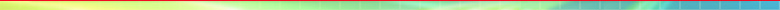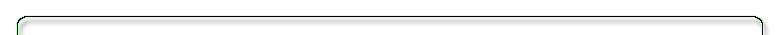### Research interests:

Currently my research has centered on questions about how the solutions of various elliptic equations, or systems of equations depend on boundary conditions.

The simplest, and prototypical, problem is Laplace's equation. The class of all solutions of Laplace's equation on a region G in space is called the class of  harmonic functions on G. A classical result is that, when G is bounded and the boundary of G is nice enough, then the Dirichlet solution map P is a compact map from L^2(bdy G) to
L^2(G). Such maps have a singular value decomposition (SVD) - an analog (and generalization) of the spectral theorem for compact linear maps of a Hilbert space to itself.

In recent years I have studied special bases of Steklov eigenfunctions of the Laplacian and the bi-Laplacian that provide orthogonal representations and SVDs of the solutions of Laplace's equations for a number of different types of boundary conditions. With students, we have found that these provide simple and good approximations of the solutions of Laplace's equations on some different regions.

I am particularly interested in the application of these ideas to various problems in classical field theories. Thus I am studying issues about approximations and representations of solutions of div-curl systems, biharmonic equations, the equations of fluid mechanics and electromagnetism.

Mathematically these issues often are connected with questions about trace spaces and trace inequalities.  If you have specific problems of this type I am happy to discuss what the possible dependence of the solutions on the boundary data is and how such dependence can be quantified.

For a listing of recent papers see Recent Publications.
For a full listing of research papers, arranged by topic, see Scientific Publications.
See also Reviews from MathSciNet.

Current Address: Department of Mathematics, PGH Building, University of Houston, Houston, Texas 77204-3008
Phone(UH): (713) 743-3500 - Fax(UH): (713) 743-3505